### Home > A2C > Chapter Ch9 > Lesson 9.3.2 > Problem9-149

9-149.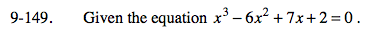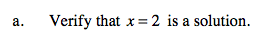Substitute 2 into the equation for every x.

8 − 24 + 14 + 2 = 0

0 = 0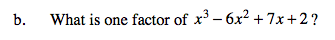Use the value for x given in problem a to calculate the factor.

(x − 2)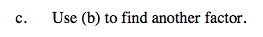Try using a generic rectangle.

(x2 − 4x −1)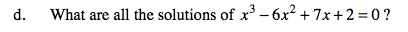See part (a) for one solution.

$\frac{4\pm\sqrt{16+4}}{2}$

Use the quadratic formula to find the other solutions in the equation x2 − 4x − 1.

$x=2, 2\pm\sqrt{5}$